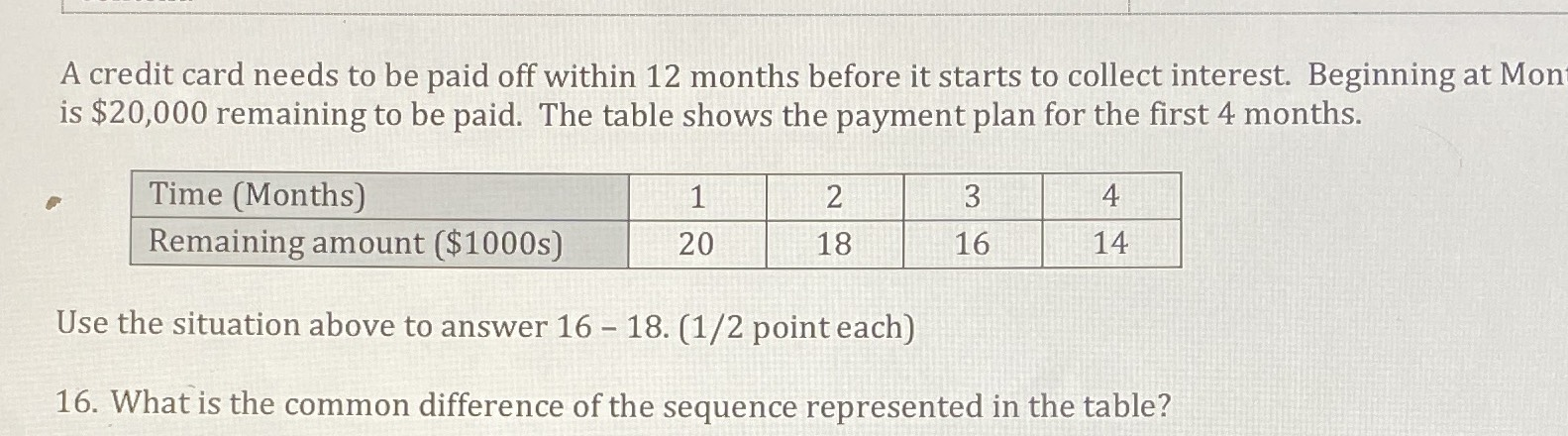### ¿Todavía tienes preguntas de matemáticas?

Pregunte a nuestros tutores expertos
Algebra
PreguntaA credit card needs to be paid off within $$12$$ months before it starts to collect interest. Beginning at Mon is $$\ 20,000$$ remaining to be paid. The table shows the payment plan for the first $$4$$ months. Use the situation above to answer $$16 - 18 . ( 1 / 2$$ point each $$)$$ 16. What is the common difference of the sequence represented in the table?